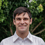This is an archived static version of the original phylobabble.org discussion site.

# [Paper] The space of ultrametric phylogenetic treesematsen

New from @alexei_drummond and his postdoc:

The space of ultrametric phylogenetic trees by Alex Gavruskin, Alexei J. Drummond

We introduce two metric spaces on ultrametric phylogenetic trees and compare them with existing models of tree space. We formulate several formal requirements that a metric space on phylogenetic trees must possess in order to be a satisfactory space for statistical analysis, and justify them. We show that only a few known constructions of the space of phylogenetic trees satisfy these requirements. However, our results suggest that these basic requirements are not enough to distinguish between the two metric spaces we introduce and that the choice between metric spaces requires additional properties to be considered.

http://arxiv.org/abs/1410.3544

I haven’t read it in detail, but it seems that the most version of the space that is most natural for time-trees (their t-space) has properties that make it mathematically difficult to analyze. The combinatorial machinery that helped out with the BHV space doesn’t help here.

Theorem 8. The problem of computing geodesics in t-space is NP-hard. We will reduce the problem of computing NNI-distance to the problem of computing geodesics in t-space, but before going on to the proof of this result, we would like to develop some intuition of why t-space is so different from both BHV and τ -space. The key property for this difference is that the cone-path is rarely a geodesic in t-space. Indeed, in both BHV and τ - space the position of two cubes can result in a cone-path being the geodesic between any pair of trees from these cubes. Particularly, the measure of the set of pairs of trees between which the cone-path is a geodesic is positive. For example, if two trees T and R have topologies with no compatible splits, then the geodesic between T and R is a cone-path . A property such as this does not present in t-space. It will follow from the observations below that the measure of the set of pairs of trees between which the geodesic is a cone-path in t-space has measure 0.

I know @cwhidden has been reading it so perhaps he’ll post some observations.